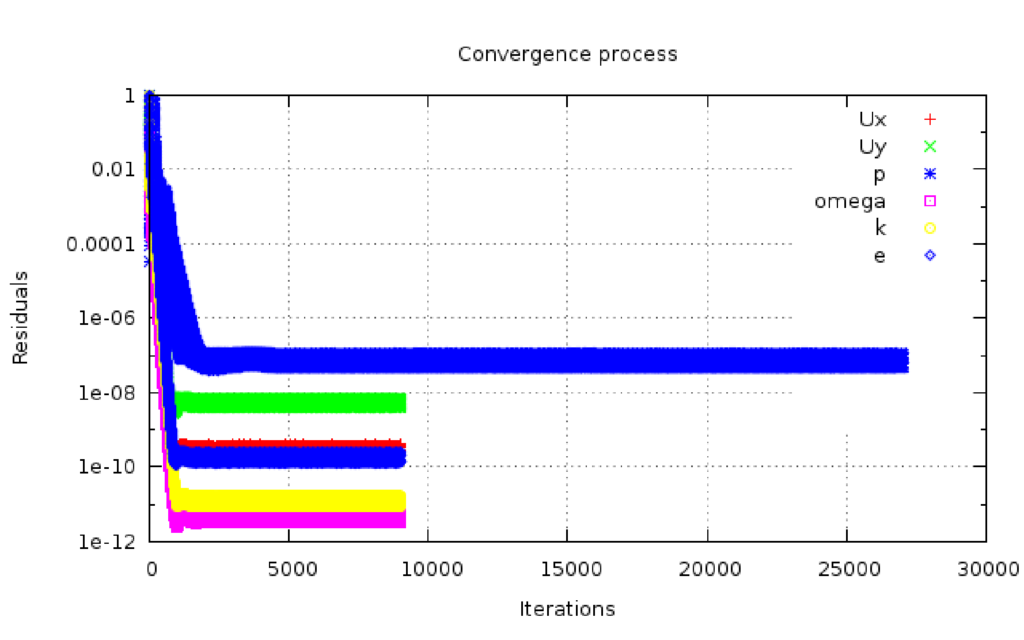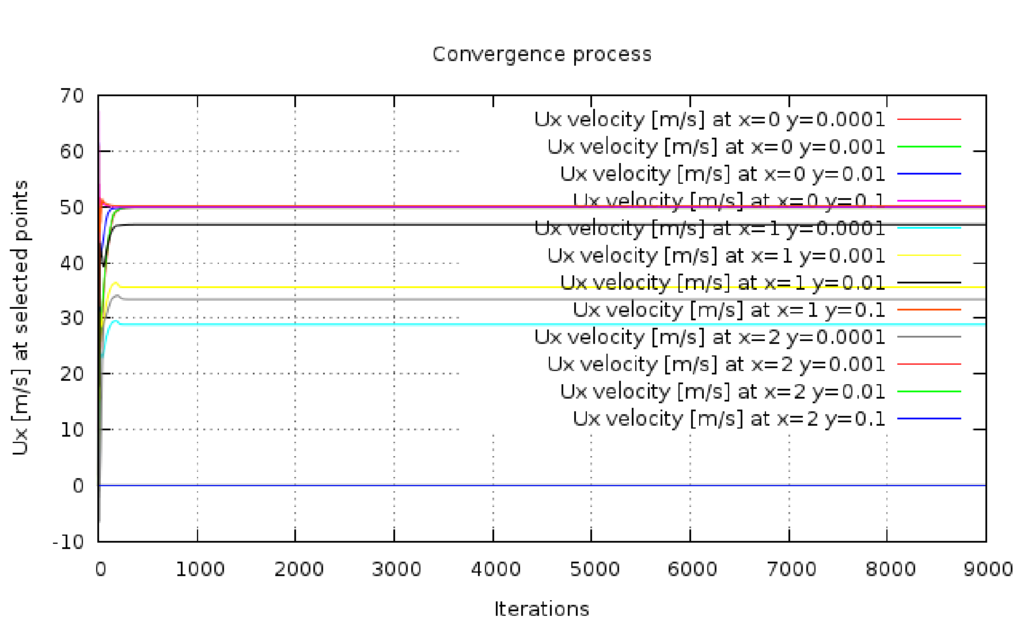This is an automatically generated documentation by LaTeX2HTML utility. In case of any issue, please, contact us at info@cfdsupport.com.

## Notes on Convergence

• before evaluating results the solution has to be perfectly converged

• always make sure the solution is fully converged

• residuals and probes at locations are useful to plot during the simulation

• probes are set via function object in controlDict

• the graph is plotted with simple script in gnuplot

• meshes having high aspect ratio cells (very thin cells) typically converge slowly in pressure. This is why with every finer mesh (perhaps higher aspect ratio) we also need to test how many iteration are needed to fully convergeFigure: The residual convergence example. (Pressure may have different number of points, because of the number of nonOrthogonalCorrectors, which is the number of how many times is pressure recomputed)Figure: The velocity convergence in certain selected points.